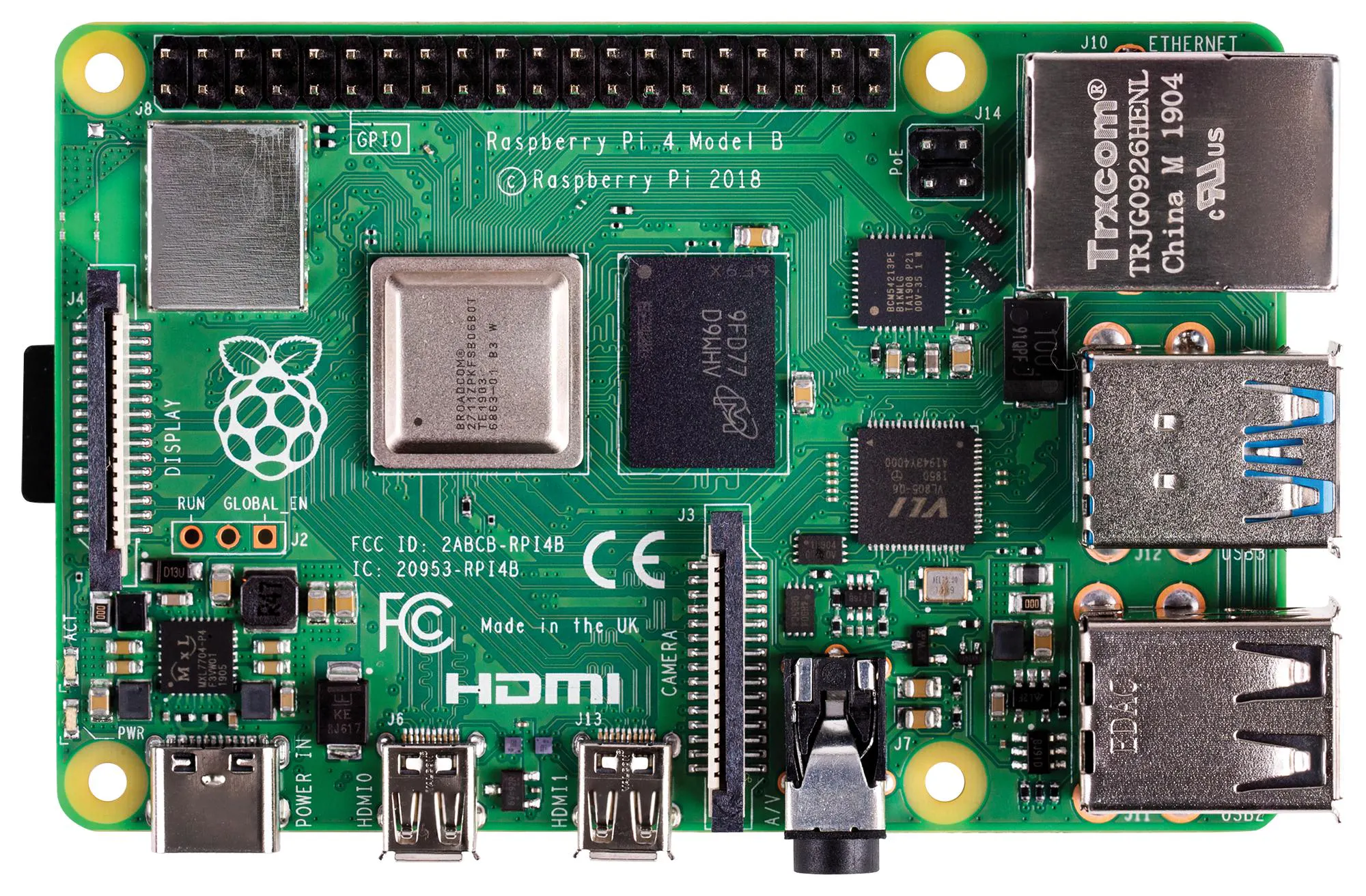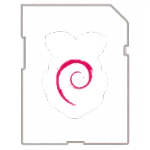# Making Art by Judging Reddit

Is the Raspberry Pi 4 powerful enough to judge Reddit? This project is all about answering the important questions.

BeginnerShowcase (no instructions)16 hours821

## Things used in this project

### Hardware components

 Screen
×1
 Picture Frame
×1Raspberry Pi 4 Model B
×1

### Software apps and online servicesRaspberry Pi RaspbianMicrosoft Azure
 Pushshift.io

## Code

### Code

Python
```import requests
import time
import json
import re
import pygame
import random

def getLatestComment():

url = "https://api.pushshift.io/reddit/search"
querystring = {"sort":"desc","sort_type":"retrieved_on","limit":"1","lang":"eng"}
response = requests.request("GET", url, params=querystring)
comment = str(d['data']['body'])
comment = comment.replace("'", "")
print('Comment: ' + comment)
return comment

def getSentimentScore(comment):

url = "https://westeurope.api.cognitive.microsoft.com/text/analytics/v2.0/sentiment"

payload = '{"documents": [{"text":"' + comment + '",  "language": "en", "id":"1"}]}'

'ocp-apim-subscription-key': "xxxxxxx",
'content-type': "text/json; charset=utf-8",
'cache-control': "no-cache"
}

try:

score =  float(d['documents']['score'])

except Exception:
score = random.random()

print('Score: '  + str(score))
return score

def run(fillScreen):

divides = 4
widthSteps = width/divides
heightSteps = height/divides
positions = []

size = (steps,steps)

for block in range(divides):

if block == 0 or block == 2:
startX = 0
else:
startX = (widthSteps * 2)

if block == 0 or block == 1:
startY = 0
else:
startY = (heightSteps * 2)

startPositions = (startX,startY)

for segment in range(divides):

if segment % 2 != 0:
posX = startX + (widthSteps * 2 - steps)
else:
posX = startX

if segment == 2 or segment == 3:
posY = startY + (heightSteps * 2 - steps)
else:
posY = startY

position = (posX,posY)
positions.append(position)

rightDown = [0,4,8,12]
rightUp =   [2,6,10,14]

leftDown = [1,5,9,13]
leftUp =   [3,7,11,15]

for i in range(120):

index = 0

if fillScreen == False:
time.sleep(2)
comment = getLatestComment()
score = getSentimentScore(comment)
randomColour = 255 * score
else:
randomColour = random.random() * 255

colour = (255,randomColour,randomColour)

for position in positions:

pygame.draw.rect(surface,colour,(position,size))
pygame.display.update()

if index in rightDown:

if index == 0 or index == 8:
if position == 420:
position = (0 , position + steps)
else:
position = (position + steps, position)

elif index == 4 or index == 12:
if position == 1320:
position = (900 , position + steps)
else:
position = (position + steps, position)

elif index in rightUp:

if index == 2 or index == 10:
if position == 420:
position = (0 , position - steps)
else:
position = (position + steps, position)

elif index == 6 or index == 14:
if position == 1320:
position = (900 , position - steps)
else:
position = (position + steps, position)

elif index in leftDown:

if index == 1 or index == 9:

if position == 450:
position = (870 , position + steps)
else:
position = (position - steps, position)

elif index == 5 or index == 13:

if position == 1350:
position = (1770 , position + steps)
else:
position = (position - steps, position)

if index in leftUp:

if index == 3 or index == 11:

if position == 450:
position = (870 , position - steps)
else:
position = (position - steps, position)

elif index == 7 or index == 15:

if position == 1350:
position = (1770 , position - steps)
else:
position = (position - steps, position)

positions[index] = position
index += 1

pygame.init()
width = 1800
height = 960
steps = 30

#Create a displace surface object
surface = pygame.display.set_mode((0, 0), pygame.FULLSCREEN)

run(True)

while True:
run(False)
```

## Credits

### 8bitsandabyte

65 projects • 48 followers
We’re Dane & Nicole, two makers that create tremendously terrible tech, which we happily share with you!